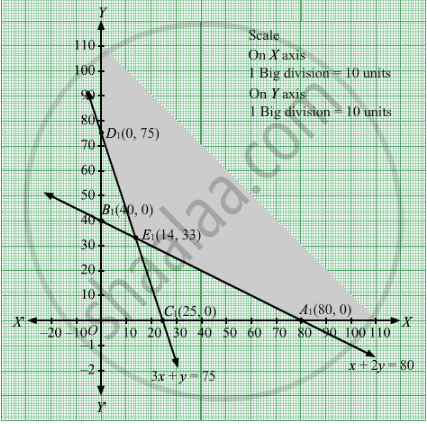# A Chemical Company Produces Two Compounds, a and B. the Units of Ingredients, C and D per Kg of Compounds a and B as Well as Minimum Requirements of C and D and Costs per Kg of a and B - Mathematics

Sum

A chemical company produces two compounds, A and B. The following table gives the units of ingredients, C and D per kg of compounds A and B as well as minimum requirements of C and D and costs per kg of A and B. Find the quantities of A and B which would give a supply of C and D at a minimum cost.

 Compound Minimum requirement A B Ingredient CIngredient D 13 21 8075 Cost (in Rs) per kg 4 6 -

#### Solution

Let x kg of compound A and y kg of compound B were produced.
Quantity cannot be negative.
Therefore, $x, y \geq 0$

 Compound Minimum requirement A B Ingredient CIngredient D 13 21 8075 Cost (in Rs) per kg 4 6 -

According to question, the constraints are

$x + 2y \geq 80$

$3x + y \geq 75$
Cost (in Rs) per kg of
compound A and compound B is Rs 4 and Rs 6 respectively.Therefore, cost of x kg of compound A and y kg of compound B is 4x and 6y  respectively.
Total cost  = Z =  $4x + 6y$

which is to be minimised.
Thus, the mathematical formulat​ion of the given linear programming problem is
Min Z =   $4x + 6y$
subject to

$x + 2y \geq 80$
$3x + y \geq 75$

$x, y \geq 0$
First we will convert inequations into equations as follows:
x + 2y = 80, 3x + y =75, x = 0 and y = 0

Region represented by x + 2y ≥ 80:
The line x + 2y = 80 meets the coordinate axes at A1(80, 0) and B1(0, 40) respectively. By joining these points we obtain the line x + 2y = 80. Clearly (0,0) does not satisfies the x + 2y = 80. So, the region which does not contain the origin represents the solution set of the inequation x + 2y ≥ 80.

Region represented by 3x + y ≥ 75:
The line 3x + y =75 meets the coordinate axes at C1(25, 0) and D1(0, 75) respectively. By joining these points we obtain the line 3x + y =75. Clearly (0,0) does not satisfies the inequation 3x + y ≥ 75. So,the region which does not contain the origin represents the solution set of the inequation 3x + y ≥ 75.

Region represented by x ≥ 0 and y ≥ 0:
Since, every point in the first quadrant satisfies these inequations. So, the first quadrant is the region represented by the inequations x ≥ 0, and y ≥ 0.

The feasible region determined by the system of constraints x + 2y ≥ 80, 3x + y ≥ 75, x ≥ 0, and y ≥ 0 are as follows.The corner points are D1(0, 75), E1(14, 33) and A1(80, 0).

The values of Z at these corner points are as follows

 Corner point Z= 4x + 6y D1 450 E1 254 A1 320

The minimum value of Z is 254 which is attained at E$\left( 14, 33 \right)$
Thus, the minimum cost is Rs 254 obtained when 14 units of compound A and 33 units of compound  B were produced.

Concept: Graphical Method of Solving Linear Programming Problems
Is there an error in this question or solution?

#### APPEARS IN

Balbharati Mathematics and Statistics 1 (Arts and Science) 12th Standard HSC Maharashtra State Board
Chapter 7 Linear Programming
Miscellaneous exercise 7 | Q 9 | Page 244
RD Sharma Class 12 Maths
Chapter 30 Linear programming
Exercise 30.4 | Q 17 | Page 52